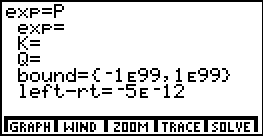# Knowledge Base

## Solution 10446: Storing and Solving Formulas on the TI-86 Graphing Calculator.

### How can I create and save a formula in the equation solver on a TI-86?

The example below shows how to solve an equation on the TI-86:

Example: Solve for K in the formula P= K^2*Q/2

Solution:

1) On the home screen, type P= K^2*Q/2
2) Press [ENTER]. The display screen will say "Done."
3) Press [2nd] [SOLVER].
4) Press [F1]. P should appear here.
5) Press [ENTER] to open the equation.
6) Move the cursor to exp= and input the value of P.
7) Move te cursor to Q= and input the value of Q.
8) Move the cursor to K= and press [F5].